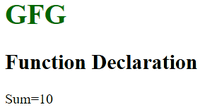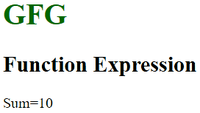Open in App
Not now

# Difference between ‘function declaration’ and ‘function expression’ in JavaScript

• Difficulty Level : Easy
• Last Updated : 09 Dec, 2022

Functions in JavaScript allow us to carry out some set of actions, important decisions, or calculations and even makes our website more interactive. Most of us coding enthusiasts know what a function is. But do we know what’s the difference between function declarations and function expressions? This article let us learn the difference between ‘function declaration’ and ‘function expression’. The similarity is both use the keyword function and the most prominent difference being the function declaration has a function name while the latter doesn’t have one.

Function Declaration:

• A function declaration also known as a function statement declares a function with a function keyword. The function declaration must have a function name.
• Function declaration does not require a variable assignment as they are standalone constructs and they cannot be nested inside a functional block.
• These are executed before any other code.
• The function in function declaration can be accessed before and after the function definition.

Syntax:

```function geeksforGeeks(paramA, paramB) {
// Set of statements
}```

Function Expression:

• A function Expression is similar to a function declaration without the function name.
• Function expressions can be stored in a variable assignment.
• Function expressions load and execute only when the program interpreter reaches the line of code.
• The function in function expression can be accessed only after the function definition.

Syntax:

```var geeksforGeeks= function(paramA, paramB) {
// Set of statements
}```

Example 1: Function Declaration

The following example illustrates a function declaration where we do the addition of two numbers.

## HTML

 `` `<``html``>`   `<``head``>` `    ``<``title``>Function Declaration` ``   `<``body``>` `    ``<``h1` `style``=``"color:green"``>GFG` `    ``<``h2``>Function Declaration`   `    ``<``script``>` `    `  `        ``// Function Declaration` `        ``function geeksforGeeks(paramA, paramB) {` `            ``return paramA + paramB;` `        ``}`   `        ``var result = geeksforGeeks(5, 5);` `        ``document.write('Sum=', result);` `    ``` ``   ``

Output:Example 2: Function Expression

The following example illustrates a function expression where we do the addition of two numbers.

## HTML

 `` `<``html``>`   `<``head``>` `    ``<``title``>Function Declaration` ``   `<``body``>` `    ``<``h1` `style``=``"color:green"``>GFG` `    ``<``h2``>Function Expression`   `    ``<``script``>` `    `  `        ``// Function Expression` `        ``var geeksforGeeks = function (paramA, paramB) {` `            ``return paramA + paramB;` `        ``}`   `        ``var result = geeksforGeeks(5, 5);` `        ``document.write('Sum=', result);` `    ``` ``   ``

Output:Difference between Function Declaration and Function Expression:

My Personal Notes arrow_drop_up
Related Articles